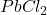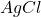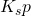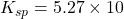There are many compounds that are slightly soluble in water known as sparingly soluble salts, such as lead chloride (), silver chloride (), Barium sulphate (), etc. When these sparingly soluble salts are dissolved in water, an equilibrium is established between the undissolved solid salt and ions of the dissolved salt.

Let us consider a sparingly soluble salt AgCl, when dissolved in water following equilibrium occurs between the undissolved solid salt and the silver and chloride ions in the saturated solution:Applying the law of chemical equilibrium, we getThe equilibrium constantis the solubility product constant. It is to be noted here that the concentration of pure solid substance remains constant, thus the concentration ofis not included in the expression.

Since the solubility of salt varies widely with temperature, the numerical value ofalso varies with temperature. The solubility of salt can easily be calculated from the solubility product constant at a given temperature.

Let us understand the relation between the solubility of salt and solubility product constant more clearly while solving a numerical problem through a video.

This video covers the solution of a numerical problem: Calculate the volume of water needed to solubilize 0.05g of Cadmium hydroxide.